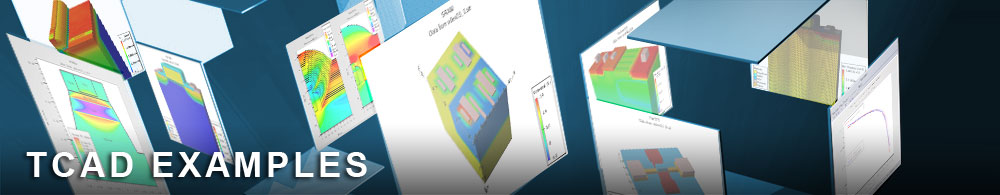Simulating a Multi-Quantum Well AlGaInP Laser Diode

## laserex05.in : Simulating a Multi-Quantum Well AlGaInP Laser Diode

Requires: Blaze/Laser/Quantum
Minimum Versions: Atlas 5.28.1.R

This example demonstrates the application of Laser to simulate a multi-quantum well laser diode. The key points of this simulation are :

• One mesh for both electrical and laser simulations
• Laser structure definition using new Atlas region syntax
• Optical and electrical material parameters specification for the chosen materials
• Application of the QWELL parameter for active layer creation and quantum well simulation
• Use of LASER statement to define laser attributes
• The same BLAZE mesh used for both electrical and laser simulations

This example is derived from published work [IEEE Journal of Quantum Electronics, VOL. 34, NO. 9, September 1998] and is reproduced as closely as possible in this simulation.

Mesh Creation

The standard Atlas mesh is used in both the electrical and laser parts of this simulation. If no specific laser mesh is created then Laser defaults to the standard Atlas mesh.

Region Creation

The REGION statements are used to define many region quantities. The material type is defined with the material parameter and the composition fractions within this material are set using the X.COMP and Y.COMP parameters appropriately.

The doping type and concentration are also defined on the region statement where the parameters acceptor and donor are used to define a uniform concentration in each region. If a non-uniform concentration is required then the DOPING statement would need to be used instead.

Material Parameters

The material statement is used to set various physical quantities for each material region in the device. The band gap, conduction and valence band density of states and band alignment are declared with the parameters eg300, NC300, NV300 and align. The material permittivity for the solution of Poisson's equation and the high frequency bulk dielectric constant are both explicity set with the parameters permittivity and epsinf. Finally, the material lifetimes taun0 and taup0 and the constant of radiative recombination COPT are defined.

Quantum Wells (QWELL)

The qwell parameter of the models statement firstly creates new material region(s) that will contain the quantum wells. Both the individual barrier width wb and the quantum well width ww need to be defined. Then a solution is found for bound state energies in the quantum well by a solution to a coupled set of Poisson / Schrodinger equations. The solution to this equation is then used in the yan model to calculate the optical gain of the quantum well.

Laser and Physical Models

The physical models may be chosen from the manual as normal and in this simulation there are 3 key models. The fermi model is chosen to correctly simulate population inversion statistics within the active region. The laser gain model chosen for the active regions is the multiple quantum well model chosen with the gainmod=4 parameter. Finally the spontaneous recombination model optr has been chosen to calculate the spontaneous recombination in the device. The spontaneous recombination is used in the photon rate equations to calculate the photon density.

The solution to the Helmholtz equation is controlled by parameters on the laser statement. On this line the rear and front reflectivities can be defined with the rf and rr parameters. The length of the laser cavity must be defined, so that the longitudinal modes can be calculated correctly, using the cavity.length parameter.

To load and run this example, select the Load button in DeckBuild > Examples. This will copy the input file and any support files to your current working directory. Select the Run button in DeckBuild to execute the example.